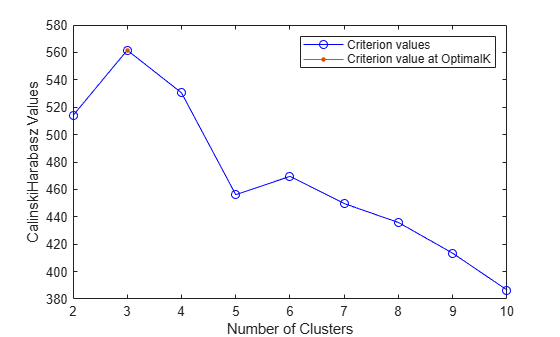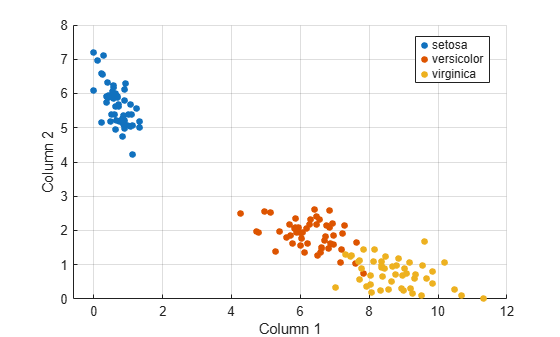Main Content

# Cluster Evaluation

This example shows how to identify clusters in Fisher's iris data.

Load Fisher's iris data set.

```load fisheriris X = meas; y = categorical(species);```

`X` is a numeric matrix that contains two petal measurements for 150 irises. `Y` is a cell array of character vectors that contains the corresponding iris species.

Evaluate multiple clusters from 1 to 10.

`eva = evalclusters(X,'kmeans','CalinskiHarabasz','KList',1:10)`
```eva = CalinskiHarabaszEvaluation with properties: NumObservations: 150 InspectedK: [1 2 3 4 5 6 7 8 9 10] CriterionValues: [1x10 double] OptimalK: 1 ```

The `OptimalK `value indicates that, based on the Calinski-Harabasz criterion, the optimal number of clusters is three.

Visualize `eva` to see the results for each number of clusters.

`plot(eva)`Most clustering algorithms need prior knowledge of the number of clusters. When this information is not available, use cluster evaluation techniques to determine the number of clusters present in the data based on a specified metric.

Three clusters is consistent with the three species in the data.

`categories(y)`
```ans = 3x1 cell {'setosa' } {'versicolor'} {'virginica' } ```

Compute a nonnegative rank-two approximation of the data for visualization purposes.

`Xred = nnmf(X,2);`

The original features are reduced to two features. Since none of the features are negative, `nnmf` also guarantees that the features are nonnegative.

Confirm the three clusters visually using a scatter plot.

```gscatter(Xred(:,1),Xred(:,2),y) xlabel('Column 1') ylabel('Column 2') grid on```Download ebook